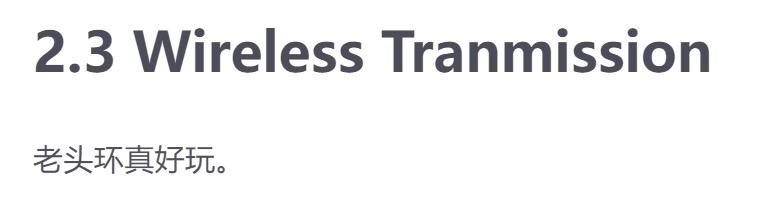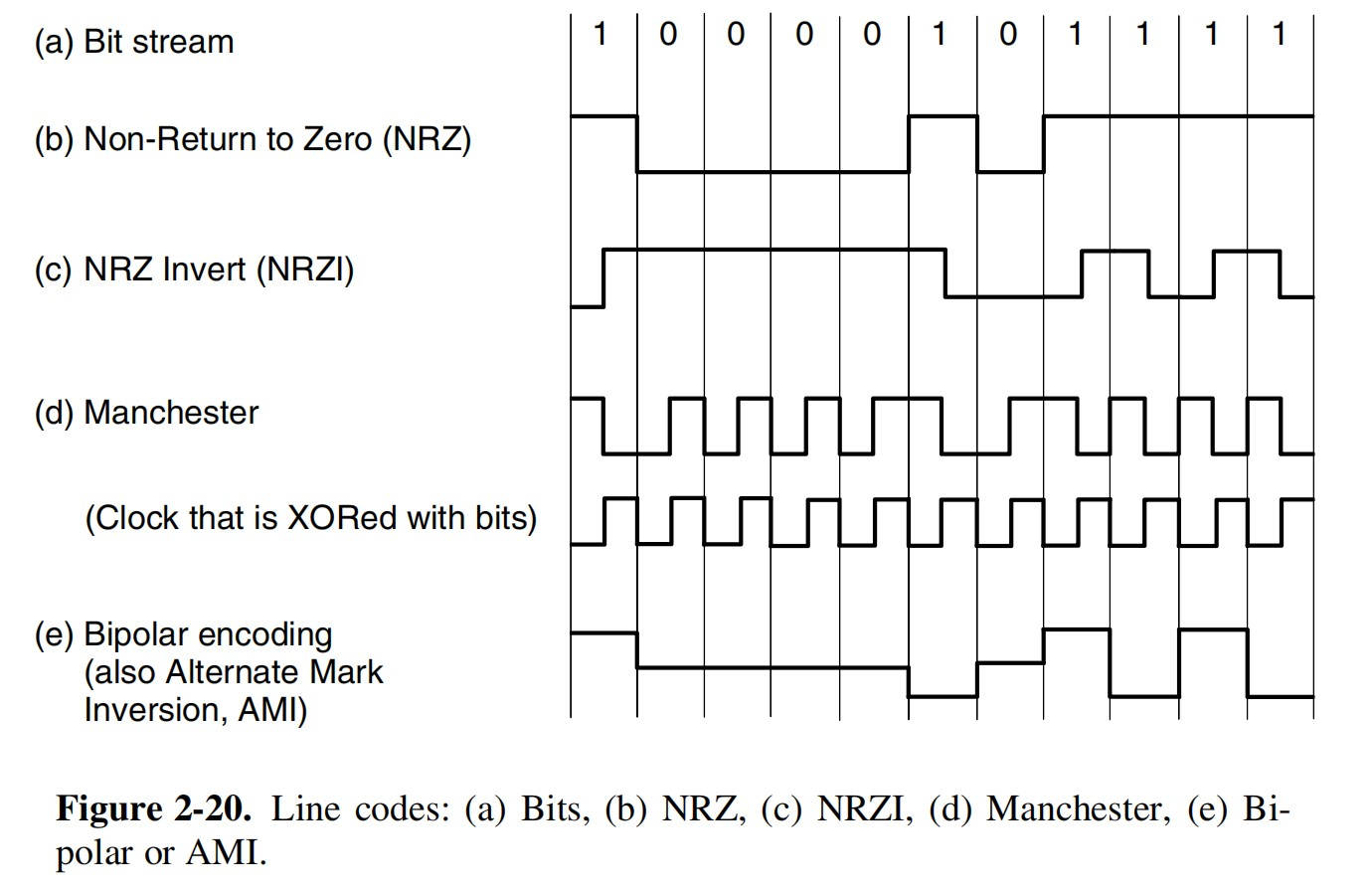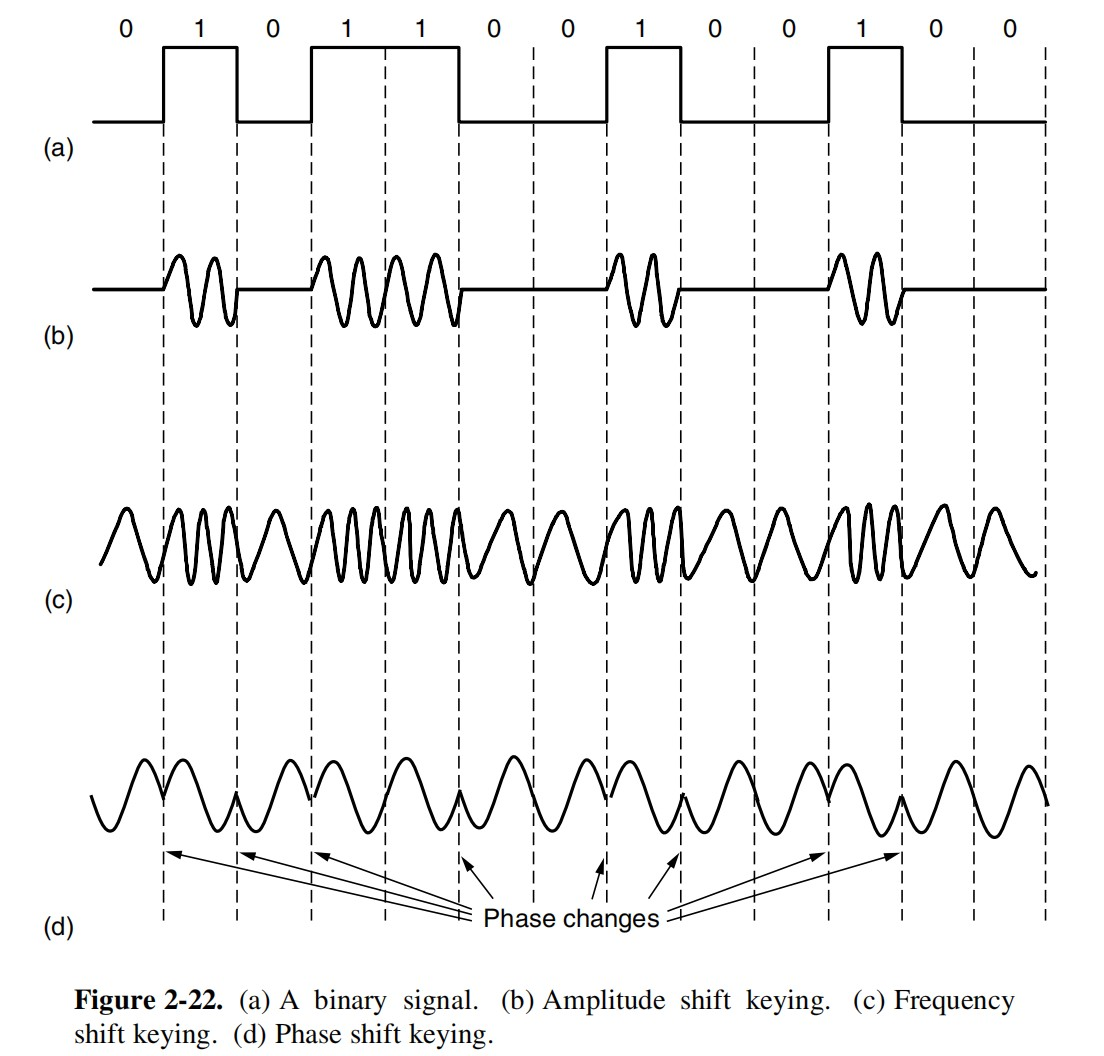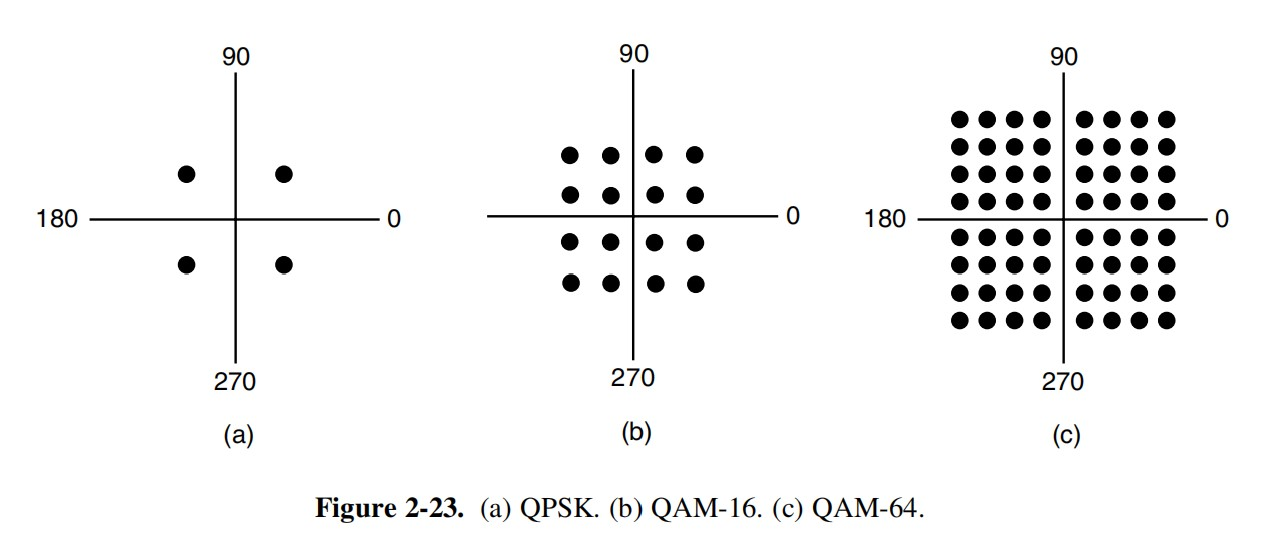## 物理层

### 香农定理

$信噪比 = \frac{信号的平均功率}{噪声的平均功率}$

$信噪比_{dB} = 10 log_{10}\frac{S}{N}$

$信道的极限传输速率 = Wlog_2(1+\frac{S}{N})$这合理吗……（来自蘑菇的笔记)

### 编码与调制ASK-调幅，FSK-调频，PSK-调相QAM-调幅+调相